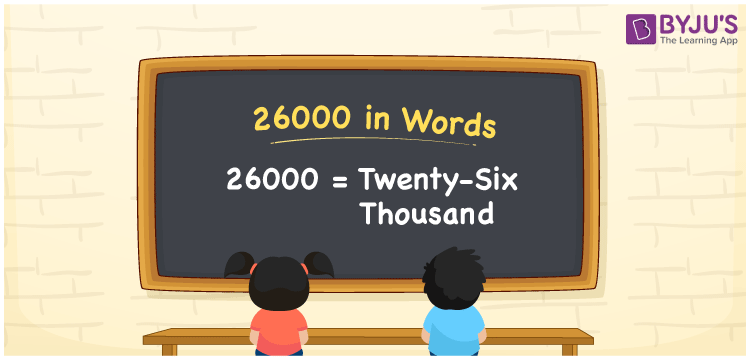# 26000 in Words

26000 in words can be written as Twenty-Six Thousand. Suppose we purchased a dishwasher worth Rs 26000, we can express this to our neighbour as, “We purchased a dishwasher worth Rs Twenty-six thousand”. We know that 26000 is a cardinal number since it denotes a specific quantity.

 26000 in words: Twenty-Six thousand Twenty-Six Thousand in numerical form 26000

## 26000 in English Words

We generally write numbers in words using the English alphabet. Hence, we can read 26000 in English as “Twenty-Six Thousand”.## How to Write 26000 in Words?

With the help of the place value chart, we can convert 26000 to words. Learn the conversion of the number 26000 to words here.

We know that 26000 has 5 digits. So, let’s make a chart that represents the place value up to 5 digits.

 Ten Thousands Thousands Hundreds Tens Ones 2 6 0 0 0

Hence, we can write the expanded form as:

2 × Ten thousand + 6 × Thousand + 0 × Hundred + 0 × Ten + 0 × One

= 2 × 10000 + 6 × 1000 + 0 × 100 + 0 × 10 + 0 × 1

= 20000 + 6000

= 26000

= Twenty-Six Thousand

Hence, 26000 in words is written as Twenty-Six Thousand.

Interesting way of writing 26000 in words

2 = Two

26 = Twenty-Six

260 = Two Hundred and Sixty

2600 = Two Thousand Six Hundred

26000 = Twenty-Six Thousand

26000 is a natural number that is succeeded by 25999 and preceded by 26001

• 26000 in words – Twenty-Six Thousand
• Is 26000 an odd number? – No
• Is 26000 an even number? – Yes
• Is 26000 a perfect square number? – No
• Is 26000 a perfect cube number? – No
• Is 26000 a prime number? – No
• Is 26000 a composite number? – Yes

## Frequently Asked Questions on 26000 in Words

Q1

### What is the correct spelling of 26000?

The correct spelling of 26000 in English words is “Twenty-Six Thousand”.
Q2

### How do you write 26000 in words on a cheque?

On a cheque, 26000 in words can be written as Twenty-Six Thousand rupees only.
Q3

### How do you say 26000 in words?

We can say 26000 in words as Twenty-Six Thousand. If this number represents the money of something, then we can express it as Twenty-Six Thousand rupees only.